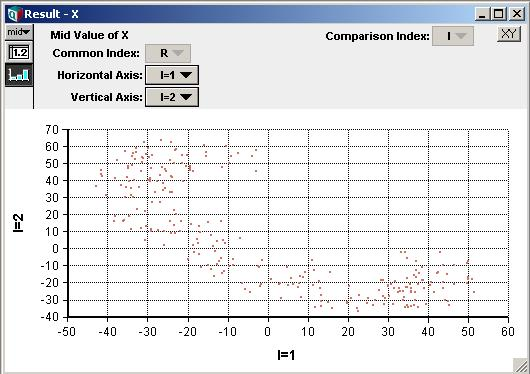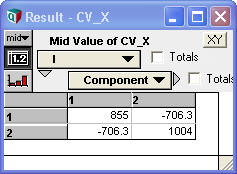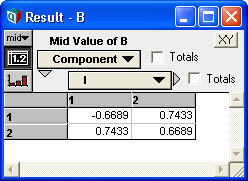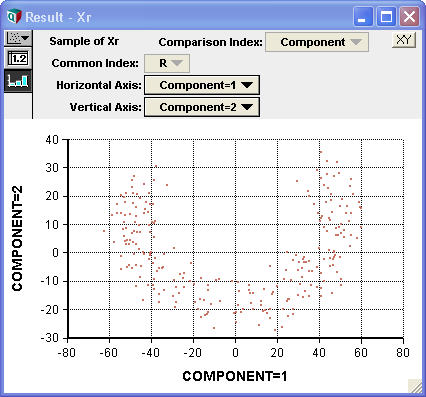# EigenDecomp

## EigenDecomp(A, I, J)

Computes the Eigenvalues and Eigenvectors of a square symmetric matrix «A» indexed by «I» and «J». Eigenvalues and Eigenvectors are also called characteristic values and characteristic vectors.

In matrix notation, when x is a row vector, then x is an Eigenvector and r the associated Eigenvalue when

A x = r x

or equivalently, when

(A - r I) x = 0

A must be symmetric to use EigenDecomp. When «A» is not symmetric, some or all of the Eigenvectors and Eigenvalues may be complex numbers.

The pairs of Eigenvalues and Eigenvectors returned are indexed by «J». If B is the result of evaluating EigenDecomp(A, I, J), then the Eigenvalues are given by

B[.item = 'value']

and the Eigenvectors are given by

#B[.item = 'vector']

Each Eigenvector is indexed by «I».

Matrix

## Testing for Definiteness

A square matrix «A» is positive definite if for for every non-zero vector x, the matrix product x'Ax > 0. The matrix «A» is positive definite if and only if all eigenvalues are positive. Also, a symmetric or non-symmetric square matrix «A» is positive definite if and only if the symmetric matrix (A+A') is positive definite. Thus the following expression tests for positive-definiteness:

Function IsPosDefinite(A: Array[I, J]; I, J: Index) :=
Min(EigenDecomp(A + Transpose(A, I, J), I, J)[.item = 'value'] > 0, J)

Square matrix «A» is positive semi-definite when x'Ax >= 0 for every vector x, which implies that the eigenvectors are all non-negative.

Function IsPosSemiDefinite(A: Array[I, J]; I, J: Index) :=
Min(EigenDecomp(A + Transpose(A, I, J), I, J)[.item = 'value'] >= 0, J)

Negative definite and Negative-semidefinite are tested for similarly:

Function IsNegDefinite(A: Array[I, J]; I, J: Index) :=
Min(EigenDecomp(A + Transpose(A, I, J), I, J)[.item = 'value']<0, J)
Function IsNegSemiDefinite(A: Array[I, J]; I, J: Index) :=
Min(EigenDecomp(A + Transpose(A, I, J), I, J)[.item = 'value'] <= 0, J)

## Principal Components

Principal components analysis is a data analysis technique that finds the rotation of your data so that the maximum variance is oriented along the first axis, the second largest component of variance is along the second axis, etc. In the rotated data, there is zero covariance along each dimension. The principal components and the accompanying variances provide a convenient description of the statistical properties of a data set. In large very high-dimensional data sets, principal component analysis can provide a means to reduce the data to a small number of dimensions by ignoring the principal components with small variance.

EigenDecomp computes the principal components from a covariance matrix. Suppose you have a dataset X[I, R], with components indexed by I, and each data point indexed by R. (Often in Analytica, R will be the Run index). To represent the Covariance matrix, another index, a copy of I is required. This new index will eventually serve to index the principal components, so it is very convenient to name it something Component and define it as CopyIndex(@I). Then the Covariance matrix of the data is obtained using:

Covariance(X, X[@I = J], R)

Call this matrix CV_X. From the covariance matrix, the variances of the principal components is obtained using:

EigenDecomp(CV_X, I, Component)[.item = 'value']

and the rotation matrix is given by

#EigenDecomp(CV_X, I, Component)[.item = 'vector']

Define B to be this rotation matrix (B is for basis). The original data can be "rotated" to its principal components, and re-centered at the origin, using:

Sum(B*(X - Mean(X, R)), I)

You could equivalently do this as a matrix multiplication using

MatrixMultiply(B, Component, I, X - Mean(X, R), I, R)

However, notice that Analytica's use of named indexes renders the Sum syntax conceptually simpler -- you know which index to sum over because it is the index that X is expressed in.

If we call this rotated data Xr, the the covariance of Xr, call it CV_Xr, computed as:

Index Component2 := Component do Covariance(Xr, Xr[Component = Component2], R)

is a diagonal matrix (i.e., zeroes everywhere except the diagonal), where the trace of the matrix, computed as

CV_Xr[Component2 = Component]

is equal to the principal component variances returned from

EigenDecomp(CV, I, Component)[.item = 'value']

### Example

Here is a scatter plot of some data, X, with indexes I and R:Covariance(X, X[@I = Component], R) →The Eigenvalues of the covariance matrix, EigenDecomp(CV_X, I, Component)[.item = 'value'], are 1613 and 188.7. You can compare these to the original variances along each axis seen in the diagonal of the covariance matrix. The largest eigenvalue has captured most of the variance.

The rotation matrix, #EigenDecomp(CV_X, I, Component)[.item = 'vector'], denoted B, isUsing this rotate the original data, using Sum(B*(X - Mean(X, R)), I), we haveThe covariance of this data, Covariance(Xr, Xr[Components = Components2], R), isThe example shown above is contained in the model Principal_components_example.ana.

## Relation to Singular Value Decomposition

The EigenDecomposition can also be computed using the SingularValueDecomp function. SingularValueDecomp decomposes a matrix «A» into a product of three matrices:

A = U W V

where «U» and «V» are orthogonal, and «W» is diagonal.

Suppose xk is the kth Eigenvector of «A» with corresponding Eigenvalue rk. By definition,

A xk = rk xk = xk rk

the second equality follows here because rk is a scalar.

Let U denote the matrix of all k eigenvectors and W denote the square diagonal matrix where each element of the diagonal is the kth Eigenvalue. By construction, U is orthonormal. Then the previous equation for all k can be written as the matrix multiplication:

A U = U W

Let V=U-1=UT, and multiplying both sides by U we get:

A = U W V

Which is exactly the decomposition computed by SingularValueDecomp. Thus, the diagonal elements of W are the eigenvalues, and the rows of U or columns of V are the eigenvectors.

## Size Limitations

Computation time scales as O(n3). The following table shows computation time of EigenDecomp on a dense random matrix running on an Intel Core I7-2600 CPU @ 3.4GHz in Analytica 64-bit.

Matrix Size CPU time
100x100 0.06 sec
500x500 0.56 sec
1000x1000 15 sec
5000x5000 12 min
10Kx10K 7.5 hours

In the 10Kx10K case, memory usage peaked at 3.5GB, which is about 2.5 times the space required to store one copy of the matrix. Since both the matrix and its result need to be stored, this indicates that there is relatively little memory overhead required.